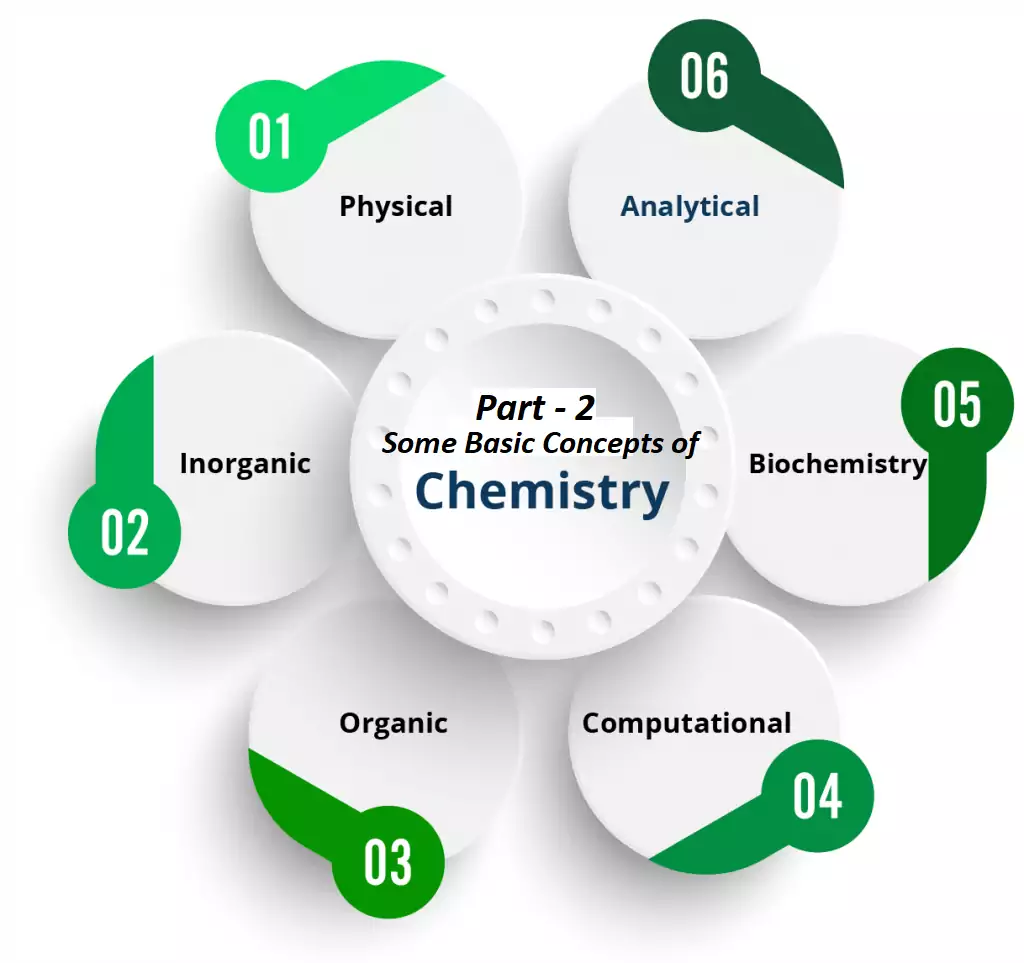# Some Basic Concepts of Chemistry Part – 2## Some Basic Concepts Chemistry Notes

Precision: It indicates how closely repeated measurements match each other.

Accuracy: It indicates how closely a measurement matches the correct or expected value.

Scientiﬁc Notation: Any number can be represented in the form where n is an exponent having positive or negative values and N can vary between 1 to 10. Expressing a number in form N × 10n, and N can vary between 1 to 10.

Signiﬁcant ﬁgures: These are meaningful digits that are known with certainty. The uncertainty is indicated by writing the certain digits and the last uncertain digit. Thus, if we write a result as 11.2 mL, we say the 11 is certain and 2 is uncertain and the uncertainty would be 1 in the last digit.
There are certain rules for determining the number of significant figures. These are:
(1). All non-zero digits are significant. For example, in 285 cm, there are three significant figures and in 0.25 mL, there are two significant figures.
(2). Zeros preceding to first non-zero digit are not significant. Such zero indicates the position of a decimal point. Thus, 0.03 has one significant figure and 0.0052 has two significant figures.
(3). Zeros between two non-zero digits are significant. Thus, 2.005 has four significant figures.
(4). Zeros at the end or right of a number are significant if they are on the right side of the decimal point; otherwise, they are not significant. For example, 0.200 g has three significant figures.
(5). Exact numbers have an infinite number of significant figures. For example, in 2 balls or 20 eggs, there are infinite significant figures since these are exact numbers and can be represented by writing an infinite number of zeros after placing a decimal i.e., 2 = 2.000000 or 20 = 20.000000

When numbers are written in scientific notation, the number of digits between 1 and 10 gives the number of significant figures. For e.g. 4.01×102 has three significant figures, and 8.256 × 10–3 has four significant figures.

Laws of Chemical Combinations:

• Law of Conservation of Mass: Antoine Lavoisier established the Law of Conservation of Mass. It states that matter can neither be created nor destroyed.

In other words, we can say that during any physical or chemical change, the total mass of reactants is equal to the total mass of products. For eg. Consider the reaction 2H2 + O2 → 2H2O
Here 4 g of H2 combines with 32 g of O2 to form 36 g of water.
Total mass of reactants = 4 + 32 = 36g. Total mass of products = 36 g

• Law of Deﬁnite Proportions: A given compound always contains the same elements in the same proportion by mass.

For Example, Carbon dioxide can be formed in the atmosphere by various methods like respiration, burning of fuels, the reaction of metal carbonates and bicarbonates with acid, etc. All these samples of CO2 contain only two elements Carbon and Oxygen combined in a mass ratio of 3:8.

• Law of Multiple Proportions: When two elements combine to form two or more compounds, then the different masses of one element, which combine with a ﬁxed mass of the other, bear a simple ratio to one another.

Hydrogen combines with oxygen to form two compounds – water and hydrogen peroxide.    Hydrogen + Oxygen → Water
2g                   16g          18g

Hydrogen + Oxygen → Hydrogen Peroxide
2g                 32g                34g

Here, the masses of oxygen (i.e. 16 g and 32 g) which combine with a fixed mass of hydrogen (2g) bear a simple ratio, i.e. 16:32 or 1: 2.

• Gay Lussac’s Law: Under similar conditions of temperature and pressure whenever gases combine together, they do so in terms of volume. e.g.,
2 H2 (g)  +  O2 (g)  →  2 H2O (g)

2 vol         1 vol           2 Vol     (at same T, P)

• Avogadro’s Law: Under similar conditions of temperature and pressure equal volume of all gases contains an equal number of molecules.

For example: If we take 10L each of NH3, N2, O2, and CO2 at the same temperature and pressure, all of them contain the same number of moles and molecules.

Dalton’s Atomic Theory: In 1808, Dalton published ‘A New System of Chemical Philosophy’ in which he proposed the following:

• Matter consists of indivisible atoms.
• All the atoms of a given element have identical properties including identical mass. Atoms of different elements differ in mass.
• Compounds are formed when atoms of different elements combine in a fixed ratio.
• Chemical reactions involve the reorganization of atoms. These are neither created nor destroyed in a chemical reaction.